Work

Workers works 5 days a week and has 21 days of vacation a year. Calculate how many days is out of work and express it as a percentage. Consider that a year has 52 weeks.

Result

n =  239
n =  65.7 %

Solution:Leave us a comment of this math problem and its solution (i.e. if it is still somewhat unclear...):Be the first to comment!To solve this verbal math problem are needed these knowledge from mathematics:

Our percentage calculator will help you quickly calculate various typical tasks with percentages.

Next similar examples:

1. Clock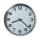How many hours are 15 days?
2. Time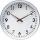16.2 days ..... how many hours is it?
3. Hours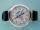How many hours is 9 days?
4. HoursThe lesson lasts 45 minutes. For the week, students have 18 lesson hours. How many are the actual hours?After two hours and 40 minutes the job is finished. Compared to last year we were 40 minutes faster. What is the percentage increase to our performance?
6. A trip to the peak Dry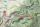Calculate what percentage is rising trip from Strečno to peak Suchý (Dry peak) longer than the descent back. Team trio of tourists (Palo, Peter and Andrew) rise took 4:21 and descent 3:08.
7. Seeds 2How many seeds germinated from 1000 pcs, when 23% no emergence?
8. Hr to minSue biked to school in 5/12 of an hour. How many minutes did it take her to ride to school?
9. Tank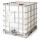How many minutes does it take to fill the tank to 25 cubic meters of water filled 150hl per hour?
10. Nightingale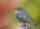Nightingale sang five seconds for 5 hours and 5 minutes. How many seconds were missing to sing 10 hours?
11. Tram lines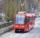Trams of five lines driven at intervals of 5,8,10,12 and 15 minutes. At 12 o'clock come out of the station at the same time. About how many hours again all meet? How many times have earch tram pass for this stop?
12. ClassIn 7.C clss are 10 girls and 20 boys. Yesterday was missing 20% of girls and 50% boys. What percentage of students missing?
13. Addition of Roman numbersAdded together and write as decimal number: LXVII + MLXIV
14. IronIron ore contains 57% iron. How much ore is needed to produce 20 tons of iron?
15. Videotape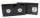Viera bought a videotape on which you can record programs with a total length of 240 minutes. She recorded a sci fi movie 1 hour and 28 minutes long, five ten-minute sessions "aerobics at home." Can she fits on the tape even film of Robin Hood who takes a
16. Minutes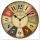Determine the difference in minutes: T1 = 2 3/20 h T2 = 2.3 h
17. TimeageSeven times of my age is 8 less than the largest two-digit number. How old I am?